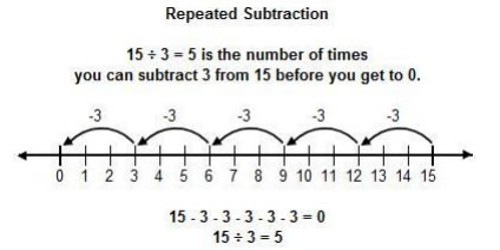Dividing by Repeated Subtractions

The result of division is to separate a group of objects into several equal smaller groups. The starting group is called the dividend. The number of groups that are separated out is called the divisor. The number of objects in each smaller group is called the quotient.

The results of division can be obtained by repeated subtraction. If we are separating 24 objects into 6 equal groups of four, we would take (or subtract) four objects at a time from the large group and place them in 6 equal groups. In mathematical terms this would be: 24-4-4-4-4-4-4.Explanation:

Division has four different components. The number we are dividing by is called the divisor. The number we are dividing into is called the dividend. The number of times the divisor fits into the dividend is called the quotient. Lastly, whatever is left over is called the remainder.

We can use repeated subtraction when faced with a division problem to find the quotient and the remainder. Suppose you have 33 pieces of candy to pass out to 5 people. This represents the division problem 33 / 5. You want each person to get an equal amount of candy, so you decide to give 5 pieces of candy at a time (one piece each), until you run out or you don’t have enough to give each of the 5 people another piece. Notice that each time you give out 5 pieces of candy, you have to subtract 5 from the total left over, and each person gets one piece of candy. Let’s see what happens.

• (33 – 5) = 28 Each person now has 1 piece of candy, and there are 28 more to pass out.
• (28 – 5) = 23 Each person now has 2 pieces of candy, and there are 23 more to pass out.
• (23 – 5) = 18 Each person now has 3 pieces of candy, and there are 18 more to pass out.
• (18 – 5) = 13 Each person now has 4 pieces of candy, and there are 13 more to pass out.
• (13 – 5) = 8 Each person now has 5 pieces of candy, and there are 8 more to pass out.
• (8 – 5) = 3 Each person now has 6 pieces of candy, and there are 3 more to pass out.

At this point, you don’t have enough to pass out another piece to each of the people, so you stop there. Each person got 6 pieces of candy, and there are 3 left over. This tells us that when we divide 33 / 5, we get a quotient of 6 and a remainder of 3.

Information Source: